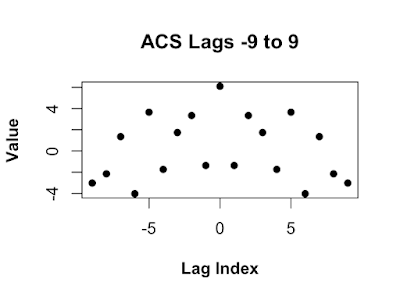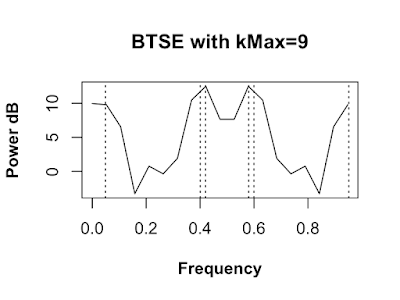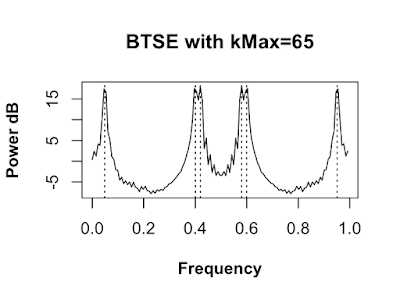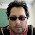## Wednesday, June 26, 2019

### Blackman-Tukey Spectral Estimator in R

Blackman-Tukey Spectral Estimator in R!

There are two definitions of the power spectral density (PSD). Both definitions are mathematically nearly identical and define a function that describes the distribution of power over the frequency components in our data set. The periodogram PSD estimator is based on the first definition of the PSD (see periodogram post). The Blackman-Tukey spectral estimator (BTSE) is based on the second definition. The second definition says, find the PSD by calculating the Fourier transform of the autocorrelation sequence (ACS). In R this definition is written as

```PSD <- function(rxx) {
fft(rxx)
}
```

where fft is the R implementation of the fast Fourier transform, rxx is the autocorrelation sequence (ACS), the k'th element of the ACS rxx[k] = E[xx[k]], k -infinity to +infinity, and E is the expectation operator. The xx in rxx[k] is a reminder r is a correlation between x and itself. The rxx[k]s are sometimes called lags. The ACS has the propriety that rxx[-k]=rxx[k]*, where * is the complex conjugate. In the post, we will only use real numbers and I'll drop the * from here forward.

So, to find the PSD we just calculate rxx and take its fft! Unfortunately, in practice, we cannot do this. Calculating the expected value requires the probability density function (PDF) of x, which we don't know and we need an infinite amount of data, which we don't have. So, we can't calculate the PSD: we're doomed!

No, we are not doomed. We can't calculate the PSD, but we estimate it! We can derive an estimator for the PSD from the definition of the PSD. First, we replace rxx with an estimate of rxx. We replace the expected value, which gives the exact rxx, with an average, which gives us an estimate of rxx. The E[xx[k]] is replaced with (1/N)(xx[1+k]+xx[2+k]+...+x[N-1-k]x[N-1]+x[N-k]x[N]), where N is the number of data samples. For example; if k=0, then rxx[k]=(1/N)*sum(x*x). In R code the estimate is written as

```lagEstimate <-function(x,k,N=length(x)){
(1/N)*sum(x[1:(N-k)]*x[(k+1):N])
}
```

If we had an infinite amount of data, N=infinity, we could use lagEstimate to estimate the entire infinite ACS. Unfortunately we don't have an infinite amount of data, even if we did it wouldn't fit into a computer. So, we can only estimate a finite amount of ASC elements. The function below calculates lags 0 to kMax.

```Lags <-function(x,kMax) {
sapply(0:kMax,lagEstimate,x=x)
}
```

Before we can try these functions out we need data. In this case the data came from a random process with the PSD plotted in the figure below. The x-axis is normalized frequency(frequency divided by the sampling rate). So, if the sampling rate was 1000 Hz, you could multiply the normalized frequency by 1000 Hz and then the frequency axis would read 0 Hz to 1000 Hz. The y-axis in in dB (10log10(amplitude)). You can see six large sharp peaks in the plot and a gradual dip towards 0 Hz and then back up. Some of the peaks are close together and will be hard to resolve.The data produced by the random process is plotted below. This is the data we will use through this post. The data is posted here: x.RDataLet's calculate the the ACS up to the 5th lag using the data.

```Lags(x,kMax=5)
```
```##   6.095786 -1.368336  3.341608  1.738122 -1.737459  3.651765
```

A kMax of 5 gives us 6 lags: {r, r, r, r, r, r}. These 6 lags are not an ACS, but are part of an ACS.

We used Lags to estimate the positive lags up to kMax, but the ACS is even sequence, r[-k]=r[k] for all k. So, let's write a function to make a sequence consisting of lags from r[-kMax] to r[kMax]. This is a windowed ACS, values outside of the +/- kMax are replaced with 0. Where it won't cause confusion, I'll refer to the windowed ACS, as the ACS.

```acsWindowed <- function(x,kMax,Nzero=0){
rHalf <- c(Lags(x,kMax),rep(0,Nzero))
c(rev(rHalf[2:length(rHalf)]),rHalf)
}
```

Let's try this function out.

```acsW <- acsWindowed(x,9)
```

In the figure below you can see the r lag, the maximum, is plotted in the middle of the plot.The ACS in the figure above is how the ACS is usually plotted in textbooks. In textbooks the sum in the Fourier transform ranges from -N/2 to (N-1)/2. So, the r lag should be in the center of the plot. In R the sum in the Fourier transform ranges from 1 to N, so the 0'th lag has to be first. We could just make the sequence in R form, but it is often handy to start in textbook from and switch to R form. We can write a function to make switching from textbook to R easy.

```Textbook2R <- function(x,N=length(x),foldN=ceiling(N/2)) {
c(x[foldN:N],x[1:(foldN-1)])
}
```

Notice in the figure below the maximum lag r, is plotted at the beginning.Let's imagine we have an infinite amount of data and used it to estimated and infinite number of ACS lags. Let's call that sequence rAll. We make a windowed ACS by setting rW=rAll*W, where W=1 for our 9 lags and 0 everywhere else. W is called the rectangular window, because, as you can see in the plot below, it's plot looks like a rectangle. By default when we estimate a finite number of lags we are using a rectangular window.

```W <- c(rep(0,9),rep(1,9),rep(0,9))
```The reason we can not use a rectangular window is its Fourier Transform is not always positive. As you can see in the plot below there are several values below zero, indicated with dotted line. Re() functions removes some small imaginary numbers due to numerical error, some imaginary dust we have to sweep up.

```FFT_W <- Re(fft(Textbook2R(W)))
```Even though the fft of the ACS rAll is positive , the produce rAll and a rectangular window might not be positive! The Bartlett window is a simple window whos fft is positive.

```BartlettWindow <- function(N,n=seq(0, (N-1)))  {
1 - abs( (n-(N-1)/2) / ( (N-1)/2) )
}
Wb <- BartlettWindow(19)
```

As you can see in the plot below the Fourier transform of the Bartlett window is positive.

```WbFft <- Re(fft(Textbook2R(Wb)))
```## Calculating the BTSE with R

Now that we can estimate the ACS and window our estimate, we are ready to estimate the PSD of our data. The BTSE is written as

```Btse <- function(rHat,Wb) {
Re(fft(rHat*Wb))
}
```

Note the Re() is correcting for numerical error.

In the first example we use a 19 point ACS lag sequence.

```rHat   <- Textbook2R(acsWindowed(x,kMax=9))
Wb     <- Textbook2R(BartlettWindow(length(rHat)))
Pbtse9 <- Btse(rHat,Wb)
```

In the figure below is the BTSE calculated with a maximum lag of 9. The dotted lines indicate the locations of the peaks in the PSD we are trying to estimate. The estimate with a maximum lage of only 9 produces a poor estimate.We calculate a new estimate with a maximum lag of 18.

```rHat    <- Textbook2R(acsWindowed(x,kMax=18))
Wb      <- Textbook2R(BartlettWindow(length(rHat)))
Pbtse18 <- Btse(rHat,Wb)
```

The next estimate is made with a maximum lag of 18. This estimate is better, the peaks around 0.4 and 0.6 are not resolved. We still need to increase the maximum lag.Finally we increase the maximum lag to 65 and recalculate the estimate.

```rHat    <- Textbook2R(acsWindowed(x,kMax=65))
Wb      <- Textbook2R(BartlettWindow(length(rHat)))
Pbtse65 <- Btse(rHat,Wb)
```

This finial estimate is very good. All six peaks are resolved and the location of our estimated peaks are very close to the true peak locations locations.## Final Thoughts

Could we use 500 lags in the BTSE? In this case we could, since we have a lot of data, but the higher lags get estimated with less data and therefore have more variance. Using the high variance lags will produce a higher variance estimate.

Are there other ways to improve the BTSE other than using more lags? Yes! There are a few other ways. For instance, we could zero pad the lags. Basically add zeros to the end of our lag sequence. This will make the fft, in the BTSE estimator, evaluate the estimate at more frequencies and we will be able to see more details in the estimated PSD.

Also keep in mind there are other PSD estimation methods that do better on other PSD features. For instance, if you we more interested finding deep nulls rather than peaks, a moving average PSD estimation would be better.

1.Interesting article, but I cannot reproduce your results. You should publish or reference the data that you use and how you create the plots so that one can follow the arguments. For example in "Lags(x,kMax=5)" x is not defined. What should it be to reproduced your results?

Thank you

2.This comment has been removed by the author.

3.Good point! I put the data here: https://github.com/carbone1853/GetYourDataOn
The data you want is x.RData
Thank you!